# Cyclical Learning Rates — Hyper parameter tuningSource: Roy’s Bikes

Neural networks are defined by connection weights and topology. While topology is usually static, the connection weights are constantly adjusted and varied to facilitate the production of desired output.

In the course of training a neural network, the connection weights are iteratively adjusted. This adjustment is determined by the resultant loss, activation function and the learning rate.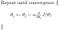alpha is the learning rate.

Years of research has consistently improved all of them. One particular paper [ Leslie N. Smith] boasts of an algorithm that improves upon the Learning Rate, rivaling adaptive optimizers and is wildly successful.

Gradient descent works like an oscillating pendulum, where the learning rate is analogous to the acceleration of the bob. We want the bob, settled at the lowest point. This is an implausible dream, unless we dampen the acceleration of the bob with drag (Friction by air).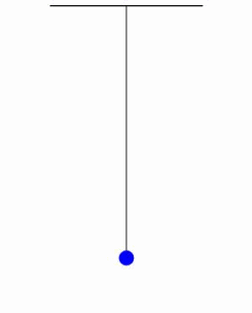Oscillating Pendulum

Similarly, neural networks will never obtain the optimal connection weights unless the learning rate is diminished. Numerous learning algorithms have incorporated adaptive learning which reduces the learning rate as the network approaches optima.

It makes sense to minimize the learning rate. But, Cyclical Learning rate is a novelty in this field as the learning rate, rather than diminishing is made to continually fluctuate periodically between a minimum and a maximum value.

# How it works

Contrary to intuition, cyclically varying the learning rate, though it might produce higher errors in the beginning has an overall improvement in results. This is primarily attributed to the ease with which it scales across saddle points during gradient descent.

Saddle points are regions where the training of the network reaches a plateau. This gives an illusion of reaching a local minima, but upon further training, improved accuracy can be obtained.

Isn’t it great? Saddle points no longer scares us anymore. Since this technique involves periodic increase in learning rate, we can escape saddle points much faster during gradient descent, saving time and resources.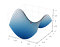Source: Wikipedia | Looks like a saddle, doesn’t it?

# Computing learning rate

The learning rate depends upon the epoch and step size. Epoch is the total number of training examples divided by the training batch size (Batch gradient descent computes gradient and updates weights for batches of training examples instead of computing the gradient for all the examples at once). Step size is experimentally determined to be 2 to 10 times the number of iterations in an epoch (Batch size).

cycle = floor ( 1 + epochCounter / ( 2 ∗ stepsize) )

x = abs ( epochCounter / stepsize − 2∗cycle + 1 )

lr = base_lr+ ( max_lr − base_lr) ∗ max ( 0 , (1−x ) )

Here, base_lr is the specified lower/base learning rate, epochCounter is the number of epochs of training, and lr is the computed learning rate and max_lr is the maximum learning rate applicable. This particular policy is known as triangular.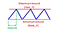Source: paper | Triangular Policy

# LR range test

We pretty much have everything required to implement our very own Cyclical learning rate algorithm. However, we are yet to determine the upper and lower bounds of our learning rate. Thus, we shall use the epic LR range test.

This is an elaborate grid search, in which the learning rate (LR) is allowed to increase linearly between a suitable minimum and maximum value. For each value of LR, the model is trained for a single epoch and the accuracy is plotted.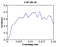Source: Paper | Accuracy plot

The learning rate at which the accuracy begins to increase should be considered as the base/minimum learning rate and the learning rate at which the accuracy begins to waver and distort into a decline is the maximum learning rate. In the figure above, since accuracy increases from the origin, we can set 0.001 as the base learning rate and since the curve begins to distort into a fall at around 0.006, we can consider it as the maximum learning rate.

# Conclusion

There are numerous optimizing algorithms to pick from, some of them use learning rate decay, others use steady ones and there are still those which utilize momentum and velocity. The effectiveness of these algorithms is situational and I’ve had instances where Adam (The most popular out these) failed, where as Stochastic Gradient Decent (SGD) worked like a charm. Thus, if you notice that your model isn’t producing desired results, don’t hesitate to tinker with the Optimizer.

# References

 Cyclical Learning Rates for Training Neural Networks by Leslie N. Smith

# Post word

Thanks for reading this article, I greatly appreciate it. It’s absolutely stunning how people produce new and creative solutions to problems. It’s always great and I’m thankful for the opportunity to learn more.

So, what do you think about Cyclical Learning rates?

Just a Geek